>>>  Go to Pro/E Tips Index  >>>
>>>  Page to Previous Pro/E Tip  >>>

September 1999

Using Equations - Part 1

Equations can be incorporated with Pro/E in a number of ways.  Over the next several months we will explore many of their uses.  To get started, this September 1999 and October 1999 Tips will explore using equations in relations - Assembly, Part, and Section Relations.

To begin, we'll make the assumption you know what RELATIONS are and how to create them.  If you do not, please consult the user guides or on-line help.  (One quick note:  In relations, lines starting with  /*  are comments.  It is a good idea to comment all your relations so you can see what you did when you come back later.  Accordingly, the relations shown in this Tip of the Month are commented.)

Assembly Relations:

There are 2 main uses for assembly relations:  first, for defining relationships in component placement; and second, for defining relationships between features of different components.

The two beams of Figure 1 demonstrate an example of where relations can define the association of components to one another.  The design intent is to maintain the two beams symmetrically about a central datum.  This is accomplished by assembling the first beam with an offset from the central datum, then assembling the second beam with an offset from the first.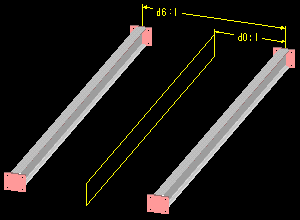The relation maintaining the beams centrally is:

/* MAKE BEAMS SYMMETRIC ABOUT CENTRAL DATUM
D0:1=D6:1/2
Notice that  d6:1,  the offset dimension for the second component, is the driving parameter.  Is this good practice to have a child drive a parent?

In this way it is fine.  The relation defines only the value of the dimension so the first component does not become a child of the second.  Why?  Only the value is dependent.  In other words, the first component does not depend on the second for its placement references, only for the value of the offset.  If the second component is removed, the value remains.

Here is another example of setting components with respect to each other.  The cross members in Figure 2 are a pattern, and the relation equations define these as being central along the main beams.  Can you write the equations for this?  (Answer in the October 1999 Tip of the Month.)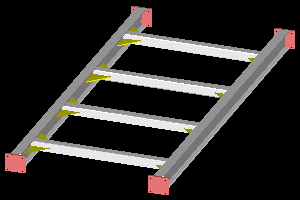The second main use of assembly relations is defining relationships between features of different components.  An example is again the cross member part in Figure 2.  The length of the protrusion (cross member) is controlled by the distance between the main beams.  Can you write this equation?

Another example is shown with the holes of Figure 3.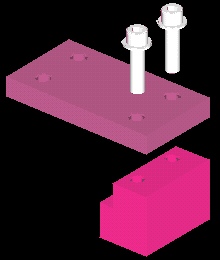The holes in one part (Figure 3) are threaded; the holes in the other part are clearance holes for bolts to pass through.  To keep the holes in line, the second set can be aligned to the first during creation, or they can be maintained positionally with relations.  Also, if we want the diameters to follow in a meaningful way, we can use a relation.  In this case, the clearance hole is defined as being 1 mm larger than the thread major diameter.  The relation equation controlling this is:

/* MAKE CLEARANCE DIA 1 MM GREATER THAN THREAD DIA
d4:6=d12:2+1
/* WHERE D4:6 IS THE CLEARANCE HOLE DIAMETER
/* WHERE D12:2 IS THE THREAD MAJOR DIAMETER
Explanation:  The  "d4"  portion of the dimension parameter is the part dimension.  The  ":2"  portion denotes the assembly member.  These numbers are assigned by Pro/E in much the same sequentially random manner as the dim parameters and internal ID's.

When relations are used in an assembly, where are the relations stored?  (A trick question perhaps, but we'll answer it in the October 1999 Tip of the Month.)

Part Relations:

Part relations are very similar to assembly relations.  They can be used to control features or dimensions with respect to each other.  A simple example is the patterned hole set shown in Figures 4A and 4B.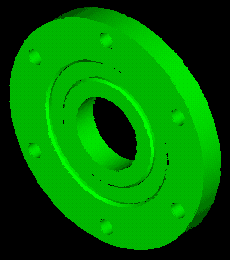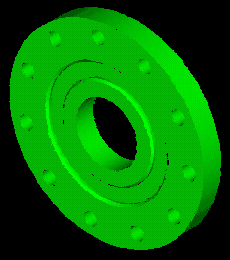Figure 4A Figure 4B

Here the hole positions are dependent on the number of holes required, and the relation maintains equal spacing around the circle.  In Figure 4A there are 6 holes, in Figure 4B it has changed to 11.  The equation maintaining this relationship is:

/* SPACE PATTERNED HOLES EQUALLY AROUND BOLT CIRCLE
D5=360/P0
Explanation:  The "p0" is the pattern number dimension parameter, in this case, 6 or 11.

The equations you create can become as complicated as you wish.  You can include many standard functions including trigonometry functions, square roots, exponents, etc..  See your help documentation for more information.

Another nice option with relations is the conditional  "IF"  statement.  Here is an exercise to try.

Exercise:  Build a UDF (User Defined Feature - see December 1998 for more information) which will automatically size the drilled hole and chamfer of Figure 3 for whatever thread diameter is defined. In other words, build a UDF to be placed into new models that will have the drilled hole, chamfer and the cosmetic thread defined to automatically update the hole and chamfer to the correct tap drill size when the thread diameter is modified. You will need to use IF statements because the difference in diameters is not always the same.Sketcher Relations:

Stay tuned, we will explore these in our October 1999 Tip of the Month.  See you then.

.

SYNTHESIS ENGINEERING SERVICES INC. :  (719) 380-1122